Looking Up Data In Tables
 As you become more proficient in Excel, you will that a very common task is looking up values in tables. For example, you may have a table of part numbers and unit prices. A simple lookup function, such as VLOOKUP or HLOOKUP can return the unit price for a specific part number. These functions are well documented in the on-line help files, and won't be discussed here.This page will describe more advanced techniques of looking up data, especially looking up data by two key values, instead of one. We'll begin with the following example. In this example, both column E and row 8 have the 'key' values for the data values in G9:K13.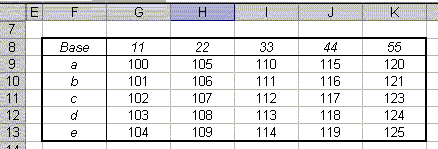Double LookupsYou can't use the VLOOKUP function to do a double lookup -- that is, a lookup based on both the row headers (column F) and the column headers (row 8). Suppose we want to return the value with a row header value of c and a column header value of 44. We can't use either VLOOKUP or HLOOKUP in this situation, because each of these functions can search only in one direction. Instead we use the MATCH and OFFSET functions. =OFFSET(\$F\$8,MATCH(F16,\$F\$9:\$F\$13,0),MATCH(G16,\$G\$8:\$K\$8,0)) In this example, cell F16 contains the desired row header (c) and cell G16 contains the desired column header (44). This function uses OFFSET to return a cell reference a specified number of row and columns from a particular cell -- in the example this "base" cell is F8. The two MATCH functions return the row and column offsets of the data. Here, the formula MATCH(F16,\$F\$9:\$F\$13,0) returns 3, because the value c is the third value in the range \$F\$9:\$F\$13. Similarly, the function MATCH(G16,\$G\$8:\$K\$8,0) returns 4, because 44 is the fourth value in the range \$G\$8:\$K\$8 . When these values are passed into OFFSET, it returns the cell that is 3 rows and 4 columns from F8. Left Lookups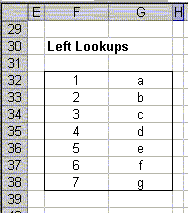Another limitation of both the VLOOKUP and HLOOKUP functions is that you can only lookup a value to the right of the key value. For example, in the range shown to the left, you can retrieve the value "c" by using VLOOKUP to search for a 3. However, the reverse is not true. It is not possible to use VLOOKUP to search for "c" and return the value 3. This sort of operation is called a left lookup, and is possible only by using the MATCH and OFFSET functions.=OFFSET(G32,MATCH(I32,\$G\$32:\$G\$38,0)-1,-1,1,1) The MATCH function tells us where in the list \$G\$32:\$G\$38 the value of I32 is, and then the OFFSET function goes to the left ( -1) to retrieve the value. Arbitrary Lookups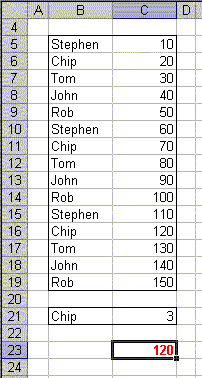If your list has duplicate values in it, VLOOKUP will always return its result based on the first match found. While this is usually useful, you may want to be able to choose which occurrence should be returned. For example, you may want to return the second or third occurrence, or perhaps the last occurrence. The following array formula will return the value from column C corresponding to a specified value in column B. That value is named in cell B21, and the occurrence is specified in cell C21.=INDEX(B5:C19,SMALL(IF(B5:B19=B21,ROW(B5:B19)-ROW(B5)+1,ROW(B19)+1),C21),2)In the example on the left, the data to search is in the range B5:C19. The value in column B to search for, Chip, is specified in cell B21, and the occurrence of that value, 3, is specified in cell C21. The result of this formula is 120, because 120 corresponds to the 3rd occurrence of Chip in column B. If you want to lookup the last occurrence of a value in the list, you can use the following array formula.=INDEX(B5:C19,SMALL(IF(B5:B19=B21,ROW(B5:B19)-ROW(B5)+1,ROW(B19)+1),COUNTIF(B5:B19,B21)),2)This is the same formula as above, but the reference to C21 has been replaced by the function COUNTIF(B5:B19,B21), which will count the number of values equal to B21 in the range B5:B19. Remember, these are array formulas, so you must press Ctrl+Shift+Enter rather than just Enter when you first enter the formula and whenever you edit it later. If you do this correctly, Excel will display the formula enclosed in curly braces { }. Closest Match Lookups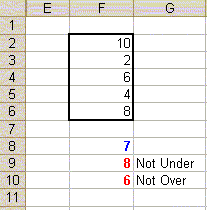The MATCH function is an important tool when working with lists of data. However, MATCH requires that the data be in sorted order unless you are searching for an exact match. If you want to find the closest value in a list to a value, the data must be sorted. You can use the INDEX and MATCH functions together to get around this limitation.The following formula will return the value from cells F2:F6 that is closest to, but not less than, the value in F8. For example, in the list shown to the left, the formula will return 8, because 8 is closest to the value 7, in F8, without exceeding it. =INDEX(F2:F6,MATCH(MIN(IF(F2:F6-F8>=0, F2:F6,FALSE)),IF(F2:F6-F8>=0,F2:F6,FALSE),0)) We can write a similar formula to return the value that is closest to, but not greater than, a given value. Referring to the list at the left, the following formula will return 6, because 6 is closest to, but not greater than, 7. =INDEX(F2:F6,MATCH(MAX(IF(F2:F6-F8<=0, F2:F6,FALSE)),IF(F2:F6-F8<=0,F2:F6,FALSE),0))Remember, these are array formulas, so you must press Ctrl+Shift+Enter rather than just Enter when you first enter the formula and whenever you edit it later. If you do this correctly, Excel will display the formula enclosed in curly braces { }.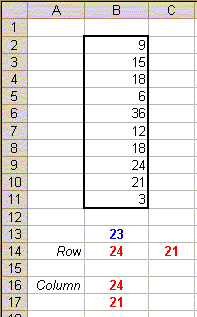The formulas shown above can be modified to find the two values in a list that come closest to a given value. Again, the data does not need to be in sorted order. For example, using the example to the left, the following formula will return 24 and 21, because these two values are closest to 23. =INDEX(B2:B11,MATCH(SMALL(ABS(B2:B11-B13), {1,2}),ABS(B2:B11-B13),0)) Since this formula returns two values, you must enter it into an array of two cells. First select the two cells which you want to contain the results, and then type the formula. Be sure to press Ctrl+Shift+Enter rather than just Enter when you enter the formula. Because this formula is in an array of cells, you won't be able to edit each cell independently. To change the formula, you must select both cells, edit the formula, and then press Ctrl+Shift+Enter when you're done. As written, the formula returns its results in to two cells in the same row, say B14:C14. If you want to return the values to two cells in the same column, say B16:B17, use the formula below. =INDEX(B2:B11,MATCH(SMALL(ABS(B2:B11-B13), {1;2}),ABS(B2:B11-B13),0)) This is the same formula as the previous formula, but the row array {1,2} is changed to a column array {1;2}. Notice that these are enclosed in curly braces {}, not parentheses.